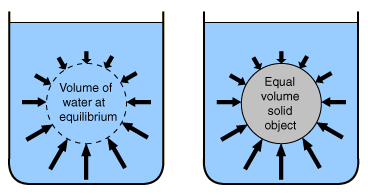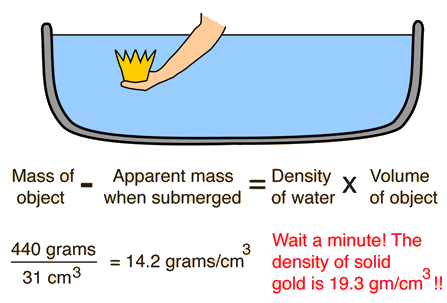# Buoyancy

Buoyancy arises from the fact that fluid pressure increases with depth and from the fact that the increased pressure is exerted in all directions (Pascal's principle) so that there is an unbalanced upward force on the bottom of a submerged object.Since the "water ball" at left is exactly supported by the difference in pressure and the solid object at right experiences exactly the same pressure environment, it follows that the buoyant force on the solid object is equal to the weight of the water displaced (Archimedes' principle).

### Applications of buoyancy.

Index

Buoyancy concepts

 HyperPhysics***** Mechanics ***** Fluids R Nave
Go Back

# Equal Volumes Feel Equal Buoyant Forces

Suppose you had equal sized balls of cork, aluminum and lead, with respective specific gravities of 0.2, 2.7, and 11.3 . If the volume of each is 10 cubic centimeters then their masses are 2, 27, and 113 gm.Each would displace 10 grams of water, yielding apparent masses of -8 (the cork would accelerate upward), 17 and 103 grams respectively.

The behavior of the three balls would certainly be different upon release from rest in the water. The cork would bob up, the aluminum would sink, and the lead would sink more rapidly. But the buoyant force on each is the same because of identical pressure environments and equal water displacement. The difference in behavior comes from the comparison of that buoyant force with the weight of the object.

 Behavior of sinking objects
Index

Buoyancy

Buoyancy concepts

 HyperPhysics***** Mechanics ***** Fluids R Nave
Go Back

# Archimedes' Principle

## Hmm! The crown seems lighter under water!The buoyant force on a submerged object is equal to the weight of the liquid displaced by the object. For water, with a density of one gram per cubic centimeter, this provides a convenient way to determine the volume of an irregularly shaped object and then to determine its density.

### What is it's density?

Index

Archimedes discussion

Buoyancy concepts

 HyperPhysics***** Mechanics ***** Fluids R Nave
Go Back

# Archimedes' Principle

## Hmm! The crown seems lighter under water!The buoyant force on a submerged object is equal to the weight of the liquid displaced by the object. For water, with a density of one gram per cubic centimeter, this provides a convenient way to determine the volume of an irregularly shaped object and then to determine its density.
Index

Archimedes discussion

Buoyancy concepts

 HyperPhysics***** Mechanics ***** Fluids R Nave
Go Back

# Archimedes' Principle

The buoyant force on a submerged object is equal to the weight of the fluid displaced. This principle is useful for determining the volume and therefore the density of an irregularly shaped object by measuring its mass in air and its effective mass when submerged in water (density = 1 gram per cubic centimeter). This effective mass under water will be its actual mass minus the mass of the fluid displaced. The difference between the real and effective mass therefore gives the mass of water displaced and allows the calculation of the volume of the irregularly shaped object (like the king's crown in the Archimedes story). The mass divided by the volume thus determined gives a measure of the average density of the object. Archimedes found that the density of the king's supposedly gold crown was actually much less than the density of gold -- implying that it was either hollow or filled with a less dense substance.Examination of the nature of buoyancy shows that the buoyant force on a volume of water and a submerged object of the same volume is the same. Since it exactly supports the volume of water, it follows that the buoyant force on any submerged object is equal to the weight of the water displaced. This is the essence of Archimedes principle.

### Application to determining density

Index

Buoyancy concepts

 HyperPhysics***** Mechanics ***** Fluids R Nave
Go Back